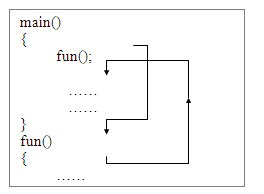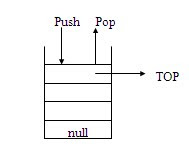/* related posts with thumb nails */

###Stack:

Stack in simple terms can be explained as a heap of objects placed one over the other.

4 The last placed object can be accessed first, and it is said to be “Last-in-First- out” (LIFO) method.

4 Stack can be implemented using both arrays and linked lists.

4 In a stack, the elements are added at the top and removed from the top i.e. both insertion and deletion take place at one end.

Some common words associated with stack:

¨ Push: Inserting an element into the stack.

¨ Pop: accessing and removing the element from the top of the stack

¨ Over-flow: when number of elements exceed the maximum size of the stack, it is said to be “ stack over flow”

¨ Under-flow: when a stack is empty, and the user is still trying to pop elements, it is called as “stack underflow”

¨ Top: refers to the top element of the stack

Applications:

Parenthesis matching: Stacks can be used to find out whether the given equation is balanced or not. It mainly checks for the matching of left and right parenthesis in an equation.

Example:

§ (A + B (x+ y) +C is not a valid expression because it misses right parenthesis

§ (A + B (x+ y) +C) is a valid expression

Convert from infix to postfix: Stacks can be used to convert an infix expression to post fix expression

Example:

§ Infix expression: (a+b)*c

§ Postfix expression: a b + c *

§ Prefix expression: * + a b c

To evaluate postfix expression: To evaluate postfix expression, stacks can be used. While evaluating, operands are stored onto a stack and popped when an operator occurs.

Example:

The postfix expression “2 3 * 6 +” gets executed as “12”

Recursion: Computer program implementing recursion uses to remember the pending jobs to be performed. It handles all the pending jobs in a stack manner.

Example:

A “Fact(n)” function written in a recursive method gets executed as follows:

Fact(3) = 3 * Fact (2)

= 3 * 2 * Fact (1)

= 3 * 2 * 1

= 3 * 2

= 6

Keeping track of function calls: To keep track of function calls, stack can be used to return to the point where it is called after executing the function. When a function is called the control shifts to the function and executes all the statements there. But, to return to the point where it is called a stack is used to remember the address of the calling statement.

Example:Computer applications that use undo features: Undo is to cancel the previous action. To remember these in LIFO order computer applications use stack.

Solving Hanoi Towers problem: Stack solves the most popular Hanoi towers problem in which an ordered set of disks must be moved into another needle that holds disks in the same order using an auxiliary needle as per some rules.

Stack is shown in the figure below:Related Topics: